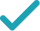Type a math problemSolve for x (complex solution)Steps Using the Quadratic Formula
Steps for Completing the Square
Steps Using Direct Factoring Method
All equations of the form can be solved using the quadratic formula: . The quadratic formula gives two solutions, one when is addition and one when it is subtraction.
This equation is in standard form: . Substitute for , for , and for in the quadratic formula, .
Multiply times .
Multiply times .
Take the square root of .
The opposite of is .
Multiply times .
Now solve the equation when is plus. Add to .
Divide by .
Now solve the equation when is minus. Subtract from .
Divide by .
The equation is now solved.
GraphGiving is as easy as 1, 2, 3
Get 1,000 points to donate to a school of your choice when you join Give With Bing
-x^{2}-x-1=0
All equations of the form ax^{2}+bx+c=0 can be solved using the quadratic formula: \frac{-b±\sqrt{b^{2}-4ac}}{2a}. The quadratic formula gives two solutions, one when ± is addition and one when it is subtraction.
x=\frac{-\left(-1\right)±\sqrt{1-4\left(-1\right)\left(-1\right)}}{2\left(-1\right)}
This equation is in standard form: ax^{2}+bx+c=0. Substitute -1 for a, -1 for b, and -1 for c in the quadratic formula, \frac{-b±\sqrt{b^{2}-4ac}}{2a}.
x=\frac{-\left(-1\right)±\sqrt{1+4\left(-1\right)}}{2\left(-1\right)}
Multiply -4 times -1.
x=\frac{-\left(-1\right)±\sqrt{1-4}}{2\left(-1\right)}
Multiply 4 times -1.
x=\frac{-\left(-1\right)±\sqrt{-3}}{2\left(-1\right)}
x=\frac{-\left(-1\right)±\sqrt{3}i}{2\left(-1\right)}
Take the square root of -3.
x=\frac{1±\sqrt{3}i}{2\left(-1\right)}
The opposite of -1 is 1.
x=\frac{1±\sqrt{3}i}{-2}
Multiply 2 times -1.
x=\frac{1+\sqrt{3}i}{-2}
Now solve the equation x=\frac{1±\sqrt{3}i}{-2} when ± is plus. Add 1 to i\sqrt{3}\approx 1.732050808i.
x=\frac{-\sqrt{3}i-1}{2}
Divide 1+i\sqrt{3}\approx 1+1.732050808i by -2.
x=\frac{-\sqrt{3}i+1}{-2}
Now solve the equation x=\frac{1±\sqrt{3}i}{-2} when ± is minus. Subtract i\sqrt{3}\approx 1.732050808i from 1.
x=\frac{-1+\sqrt{3}i}{2}
Divide 1-i\sqrt{3}\approx 1-1.732050808i by -2.
x=\frac{-\sqrt{3}i-1}{2} x=\frac{-1+\sqrt{3}i}{2}
The equation is now solved.
-x^{2}-x-1=0
Quadratic equations such as this one can be solved by completing the square. In order to complete the square, the equation must first be in the form x^{2}+bx=c.
-x^{2}-x-1-\left(-1\right)=-\left(-1\right)
Add 1 to both sides of the equation.
-x^{2}-x=-\left(-1\right)
Subtracting -1 from itself leaves 0.
-x^{2}-x=1
Subtract -1 from 0.
\frac{-x^{2}-x}{-1}=\frac{1}{-1}
Divide both sides by -1.
x^{2}+\frac{-1}{-1}x=\frac{1}{-1}
Dividing by -1 undoes the multiplication by -1.
x^{2}+x=\frac{1}{-1}
Divide -1 by -1.
x^{2}+x=-1
Divide 1 by -1.
x^{2}+x+\left(\frac{1}{2}\right)^{2}=-1+\left(\frac{1}{2}\right)^{2}
Divide 1, the coefficient of the x term, by 2 to get \frac{1}{2}=0.5. Then add the square of \frac{1}{2}=0.5 to both sides of the equation. This step makes the left hand side of the equation a perfect square.
x^{2}+x+\frac{1}{4}=-1+\frac{1}{4}
Square \frac{1}{2}=0.5 by squaring both the numerator and the denominator of the fraction.
x^{2}+x+\frac{1}{4}=-\frac{3}{4}
\left(x+\frac{1}{2}\right)^{2}=-\frac{3}{4}
Factor x^{2}+x+\frac{1}{4}. In general, when x^{2}+bx+c is a perfect square, it can always be factored as \left(x+\frac{b}{2}\right)^{2}.
\sqrt{\left(x+\frac{1}{2}\right)^{2}}=\sqrt{-\frac{3}{4}}
Take the square root of both sides of the equation.
x+\frac{1}{2}=\frac{\sqrt{3}i}{2} x+\frac{1}{2}=-\frac{\sqrt{3}i}{2}
Simplify.
x=\frac{-1+\sqrt{3}i}{2} x=\frac{-\sqrt{3}i-1}{2}
Subtract \frac{1}{2}=0.5 from both sides of the equation.
x ^ 2 +1x +1 = 0
Quadratic equations such as this one can be solved by a new direct factoring method that does not require guess work. To use the direct factoring method, the equation must be in the form x^2+Bx+C=0.
r + s = -1 rs = 1
Let r and s be the factors for the quadratic equation such that x^2+Bx+C=(x−r)(x−s) where sum of factors (r+s)=−B and the product of factors rs = C
r = -\frac{1}{2} - u s = -\frac{1}{2} + u
Two numbers r and s sum up to -1 exactly when the average of the two numbers is \frac{1}{2}*-1 = -\frac{1}{2}. You can also see that the midpoint of r and s corresponds to the axis of symmetry of the parabola represented by the quadratic equation y=x^2+Bx+C. The values of r and s are equidistant from the center by an unknown quantity u. Express r and s with respect to variable u. <div style='padding: 8px'><img src='https://opalmath.azureedge.net/customsolver/quadraticgraph.png' style='width: 100%;max-width: 700px' /></div>
(-\frac{1}{2} - u) (-\frac{1}{2} + u) = 1
To solve for unknown quantity u, substitute these in the product equation rs = 1
\frac{1}{4} - u^2 = 1
Simplify by expanding (a -b) (a + b) = a^2 – b^2
-u^2 = 1-\frac{1}{4} = \frac{3}{4}
Simplify the expression by subtracting \frac{1}{4} on both sides
u^2 = -\frac{3}{4} u = \pm\sqrt{-\frac{3}{4}} = \pm \frac{\sqrt{3}}{2}i
Simplify the expression by multiplying -1 on both sides and take the square root to obtain the value of unknown variable u
r =-\frac{1}{2} - \frac{\sqrt{3}}{2}i = -0.500 - 0.866i s = -\frac{1}{2} + \frac{\sqrt{3}}{2}i = -0.500 + 0.866i
The factors r and s are the solutions to the quadratic equation. Substitute the value of u to compute the r and s.